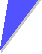# Derive numbers for v0, vD, t, and lShow that the claims made in the backbone text are actually true (for room temperature = 300 K). Use the following equations taken from the backboneFor the average velocity v0 of a particle zooming around in the crystal:
 v0   = æ ç è ö ÷ øFor the mean time t between scattering:
t  =   s · m
n · e2For the drift velocity vD
vD   =  –  E · e · t
mFor the minimal mean free path length lmin obtained for vD = 0:
lmin  =  2 · v0 · tOf course, you need numbers for the concentration n of the free carriers and for the specific conductivity sSince we are essentially considering metals, you assume for a start that you have 1 free electron per atom if you want to find a number for n. Here are a few data needed for the calculation:
Atom Density
[kg · m–3]
Atomic weight
× 1,66 · 10–27 kg
Conductivity s
× 105 [W–1 · m–1]
Conzentration Atoms n
[m–3] ???
Na 970 23 2.4
Cu 8.920 64 5.9
Au 19.300 197 4.5You may run into some trouble with the dimensions. Just look at conversions from, e.g. [eV] to [J], from W to V and A, and at the relations beween Volt, Ampere, Watts and Joule.Solution2.1.2 Ohms Law and Classical PhysicsSolution to Exercise 2.1-2